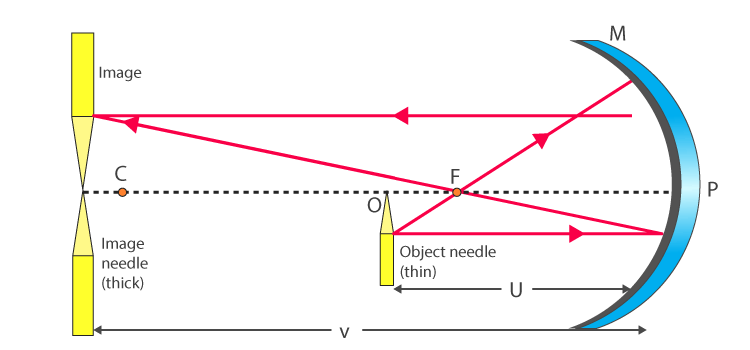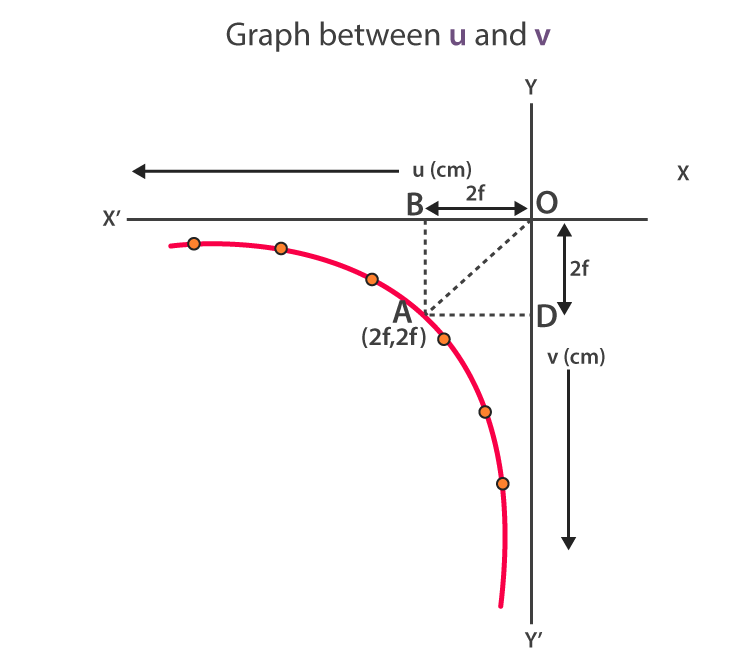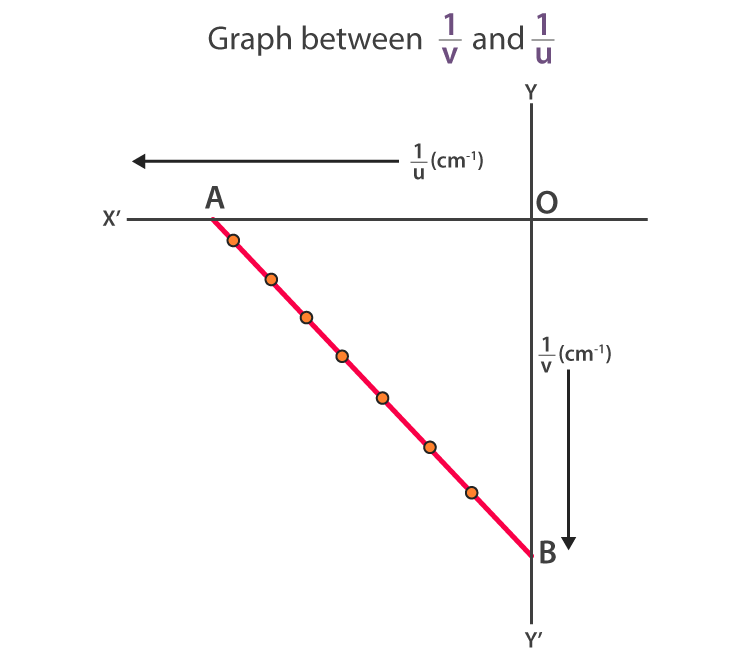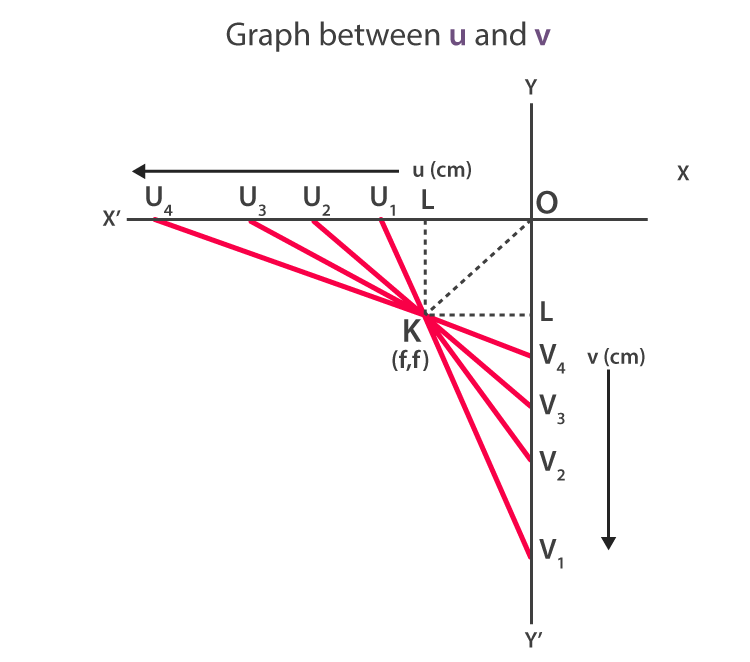# To find the value of v for different values of u in case of a concave mirror and to find the focal length

## Aim

To find the value of v for different values of u in case of a concave mirror and to find the focal length.

## Materials Required

Following are the materials required for this experiment:

• An optical bench with three uprights
• A concave mirror
• A mirror holder
• Two optical needles
• A knitting needle
• A half-metre scale

## Theory

We use mirror formula for the determination of focal length.

Mirror formula is given as:

$\frac{1}{f}=\frac{1}{v}+\frac{1}{u}$

From the above equation, we get the focal length as:

$f=\frac{uv}{u+v}$

Where,

• f is the focal length of a concave mirror
• u is the distance of object needle from the pole of the mirror
• v is the distance of image needle from the pole of the mirror

The value of f will be negative. This is according to the sign-convention, u and v have negative values.

## Ray Diagram## Procedure

To determine the rough focal length

1. Place the concave mirror in the mirror holder.
2. Take this mirror holder outside and face the mirror towards a distant building.
3. The image of the building is obtained on a wall which is painted white. Move the mirror forward and backwards to obtain a sharp image on the wall.
4. The rough focal length of the mirror is equal to the distance between the mirror and the wall.

To set the mirror

1. The placement of the fixed upright should be near zero ends of the optical bench while the other two uprights must be near the other ends.
2. Attach the mirror to the holder at the fixed upright such that the mirror faces towards the other end of the bench and surface where it is perpendicular to the length.
3. To make the mirror surface vertical, use levelling screws.
4. At the base of the mirror upright, note the position of the index mark.

To set the object needle

1. Let O be the thin optical needle which is mounted on the second upright.
2. The object needle along with the clamp is moved 1.5 times to obtain a rough focal length of the mirror.
3. To make the object needle and pole of the mirror horizontal, adjust the height of the object needle.
4. Close left eye, open right eye to see an inverted and enlarged image of the needle such that the tip of the image is at the centre of the mirror.
5. Record the index mark position at the base of the object needle.

To set the image needle

1. Attach the thick optical needle on the third upright and move it to the other end of the optical bench.
2. To make the tip of the image needle and tip of the image inline, adjust the height of the image needle by closing the left eye and opening the right eye.
3. To check if parallax exists, move the eye towards the right and see that the tips get separated.
4. Remove the parallax tip to tip.
5. At the base of the image needle upright, record the index mark.
6. To determine the index corrections, all the index marks are to be recorded as observation 2.
7. Determine the index correction for the distance between the pole of the mirror, the tip of the object needle and the image needle.
8. To get observations 2 and 1, move the object needle upright towards the mirror by 1 cm.
9. To get observations 4, 5, and 6, move the object needle away from the mirror by 1 cm.
10. Note all the observations.

## Observations

The rough focal length of the given concave mirror = …….

The actual length of the knitting needle, x = ……

Observed distance between the mirror and object needle when knitting needle is placed in between them, y = …….

Observed distance between the mirror and image needle when knitting needle is placed in between the, z = ……..

Index correction for the object distance, u = x – y = ±…….

Index correction for the image distance, v = x -z = ±…….

Table for u, v, $\frac{1}{u}$, and $\frac{1}{v}$

 Distance u (cm) 12 16 20 Distance v (cm) $y=\frac{uv}{u + v}$

## Calculations

Calculations of the focal length by graphical method

u – v graph is obtained by considering u on the x’- axis and v on y’ – axis. According to sign conventions, u and v are negative. Using the observation table, plot different points for different values of u and v. The graph obtained will be a rectangular parabola.

On either axis draw a line OA making 45° and meeting at a point A. Draw AB and AD perpendicular on x’ and y’- axis. For point A, the values of u and v will be the same. The coordinates at A is (2f, 2f) as u and v are same for a concave mirror when the object is placed at the centre of curvature. Hence, u = v = R = 2f.

### Explanation

• Applying mirror formula to point A, we get $\frac{1}{f}=\frac{1}{u}+\frac{1}{v}$ which can be written as u = v, $\frac{1}{f}=\frac{2}{u}$ or $\frac{2}{v}$ and $f=\frac{u}{2}$ or $\frac{v}{2}$.

Hence the focal length of the concave mirror is obtained from the coordinates of A.

$f=-\frac{OD}{2}=-….$

∴ Mean value of f = -…….• $\frac{1}{u}\;and\; \frac{1}{v}$ graph is obtained by considering a scale $\frac{1}{u}$ along x’ axis and $\frac{1}{v}$ along y’ axis. Both these values are negative according to sign convention. The graph obtained will be a straight line and is plotted in the third quadrant. Both the axes are cut by a straight line at 45° making equal intercepts. By measuring the distance of OA and OB, the focal length is obtained, $f=-\frac{1}{OA}=-\frac{1}{OB}=-…..\;cm$• For u-v graph, suitable scale must be selected to represent u along the X’-axis and v along the Y’-axis. Distances such as u1, u2,…. must be marked along OX’-axis and corresponding distances such as v1, v2,…..must be marked along OY’-axis. To join u1 with v1 and other corresponding points, straight lines must be drawn. All these straight lines intersect at point K. KL and KM are the perpendiculars on X’ and Y’axes.

Then -f = OL = OM

∴ f = -…… cm## Result

The focal length of the given concave mirror is determined from the following:

• Focal length is calculated as, $f=\frac{uv}{u+v}$ = ……..cm
• (u – v) graph = -……cm
• $(\frac{1}{u}-\frac{1}{v})$ graph = -………cm
• (u – v) graph = -…….cm

## Precautions

• The uprights should be vertical.
• The principal axis of the mirror should be perpendicular while the central line of the optical bench should be parallel.
• The eye should be at least 30 cm away from the needle to locate the position of the image.
• Tip to tip parallax should be removed between the needle and the image obtained from the needle.
• The tips of object and image should be at the same height when compared to the pole of the concave mirror.
• Index correction should be applied for u and v.

## Sources of Error

• Parallax removed may not be perfect.
• The uprights may not be vertical.

## Viva Questions

Q1. Define lens formula.

Ans: Lens formula is defined as the equation which explains the relation between object distance (u), image distance (v), and focal length of the lens (f).

Q2. What is the optical centre of a lens?

Ans: Optical centre of a lens is defined as the fixed point inside the lens on the principal axis through which light ray passes.

Q3. What is the principal axis of a lens?

Ans: Principal axis of a lens is the straight line passing through the centre of curvature of the lens.